Page top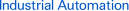# FAQ01077 of Programmable Controllers FAQ

FAQ No. FAQ01077

Question

What is the difference in the way that the BCD ADD WITHOUT CARRY instruction and BCD ADD WITH CARRY instructions are used?

﻿

The Carry Flag (CY)

Most Programmable Controllers support double-length math instructions, but for example, what should be done if the result of a BCD double-length addition operation exceeds the upper-limit of double-length data, which is 9999 9999?

BCD Double-length Data Range
0 to 9999 9999

Now for the Carry Flag (CY)!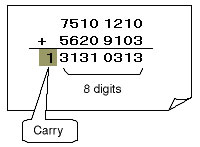Figure1

How the Carry Flag (CY) Works

The result of the addition operation shown in Figure1 cannot be expressed with only 8 digits, so an overflow (i.e., a “carry”) occurs. In this case the Carry Flag (CY) will turn ON.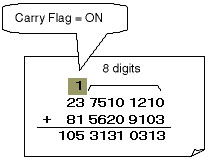Figure2

Here, the Carry Flag can be used to pass the carry to the calculation for the upper digits, enabling calculations for more than 8 digits.

The information from Figure1 and Figure2 is summarized here.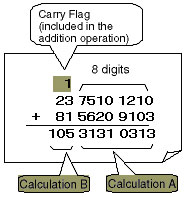Figure3

Calculation A:
Use the ADD WITHOUT CARRY instruction for rightmost digit calculations in which there is no carry from the lower digit.

Calculation B:
Use the ADD WITH CARRY instruction for upper digit calculations in which a carry from the lower digit must be considered.

The ADD WITH CARRY instruction, as shown in Figure3, is an addition instruction that includes the Carry Flag in the addition operation.

Program and Operation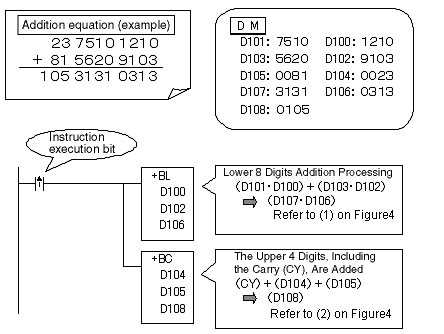2. Operation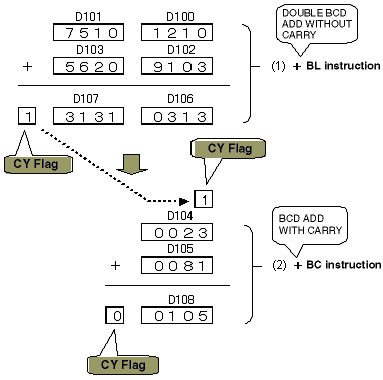Figure4

Summary

Used for rightmost digit calculations in which there is no carry signal from a lower digit.
Used for upper digit calculations in which a carry signal from the lower digit must be considered.

The ladder program above is capable of handling an addition result of 12 digits in length. However, if the result will exceed 12 digits, make the upper digit calculation instruction a Double Add Instruction, or connecting multiple operations with ADD WITH CARRY Instructions.

The CPM2A Programmable Controllers and other smaller Programmable Controllers only have the ADD WITH CARRY Instruction, so when doing calculations with lower digits, be sure to use the CLEAR CARRY (CLC) instruction to turn OFF the Carry Flag (CY) before executing Addition Instructions. If the Carry Flag (CY) is not property reset, in other steps where the Carry Flag (CY) is turned ON, arithmetic mistakes such as 1 + 1 = 3 will occur.

4.The logic works the same for Binary Addition instructions and other Math Instructions.

This document explained the carry procedure as related to the BCD Addition instruction, but the same logic applies to the Binary Addition instructions as well.
When dealing with subtraction operations, the Carry Flag (CY) and carry operation simply changes to a borrow operation and the calculation can be done in the same way.

## Recommended Products

###CJ2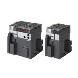Introducing the Flagship CJ2 CPU Units, with Built-in Multifunctional Ethernet Port.

###CP1E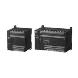Cost-effective CP1E with Enhanced Expandability for Analog and Temperature Control

###NJMotion, Logic, Safety, Vision and HMI in one